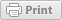Application of ANOVA for Plant Breeding: Single Marker Analysis Example using ANOVA in a Balanced Population

Plant Breeding and Genomics January 07, 2013Authors:

David M. Francis, The Ohio State University; Heather L. Merk, The Ohio State University

This module provides an example of using analysis of variance (ANOVA) to assess differences in resistance to disease due to molecular marker (treatment effect) using single marker analysis.

Introduction

This module provides an example of using analysis of variance (ANOVA) to assess differences in tomato bacterial spot resistance due to molecular marker genotype (treatment effect).

As an example, consider the case of two molecular markers, PTO and TG23, that were genotyped in a BC1 population that was phenotyped for bacterial spot disease severity.

The null hypothesis postulated was that no difference existed in disease severity between molecular marker genotypes—that is, Y = µ + error. This hypothesis was tested separately for each molecular marker.

For a review of the backcrossing procedure, three lessons are available through the University of Nebraska-Lincoln's Plant and Soil Sciences online eLibrary.

In general, the ANOVA table would look like the one that follows for each marker:

Source DF Expected Mean Square
Genotypes N-1 s2 + bs2 (G)
Marker 1 s2 + b [s2 (GQTL) + 4r (1-r) g2] + bc (1 - 2r)2 g2
Marker (Genotype) N-2 s2 + b [s2 (GQTL) + 4r (1-r) g2]
Error N (b-1) s2

where:

• b is the number of replicates
• r is the recombination fraction separating the marker from the QTL
• c is a coefficient of related to the population size
• c = N - (n12 + n22) / N
• n1 + n2 = 1, (representing the number of individuals in each marker class)
• N is the total number of individuals.
• g is the genetic effect (in backcross populations, additive and dominance effects are confounded)
• s2 (GQTL) is the part of the error variance that cannot be explained by the QTL.

When b = 1, Genotype(marker) becomes the error term. If there are repeated measures on each genotype (b > 1), the proper error term must be specified. The F test for significance is Marker/Genotype(marker) = bc (1 - 2r)2 g2

Therefore, significance of a marker depends on population size, recombination, the strength of the genetic effect relative to the error variance, and the part of the error variance that cannot be explained by the QTL.

Data Analysis

In this example, for both molecular markers, treatment effect has p < 0.0001.

Interpretation: In this example, the null hypothesis—that disease severity does not differ between the marker classes—can be rejected.

If the null hypothesis can not be rejected, there is no evidence in the experiment that either r = 0.5 (no genetic linkage) or 2g = (a+d) = 0 (there is no genetic effect).

In a simple linear model, the R2 value (R-Square in the SAS ouput) can be interpreted as a measurement of the proportion of phenotypic variation explained by the QTL. In this example, TG23 explains 77% of the observed variation (p < 0.001) while PTO explains 40.2% of the observed variation (p < 0.001).

Funding Statement

Development of this page was supported in part by the National Institute of Food and Agriculture (NIFA) Solanaceae Coordinated Agricultural Project, agreement 2009-85606-05673, administered by Michigan State University. Any opinions, findings, conclusions, or recommendations expressed in this publication are those of the author(s) and do not necessarily reflect the view of the United States Department of Agriculture.

PBGworks 905

Welcome

This is where you can find research-based information from America's land-grant universities enabled by eXtension.orgThis work is supported by the USDA National Institute of Food and Agriculture, New Technologies for Ag Extension project.